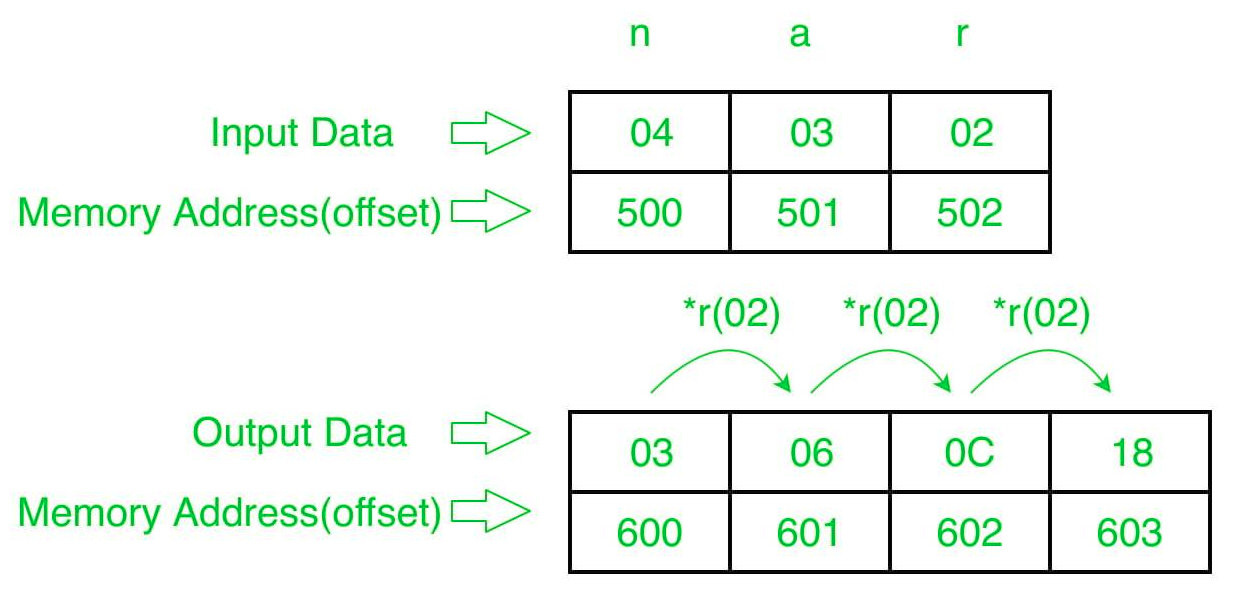# 8086 program to generate G.P. series of n numbers

• Last Updated : 19 Jun, 2018

Problem – Write a program in 8086 microprocessor to generate G.P (geometric progression) series of n numbers (numbers will be of 8-bit only), where size “n” is stored at offset 500 and the first number(a) is stored at offset 501 and store the common ratio is stored at offset 502 .Store the series into offset 600.

Example –Algorithm –

1. Store 500 to SI and 600 to DI Load data from offset 500 to register CL and set register CH to 00 (for count).
2. Increase the value of SI by 1.
3. Load first number(value) from next offset (i.e 501) to register AL.
4. Store the value of register AL to memory offset DI.
5. Increase DI by 1.
6. Decrease the CL by 1.
7. Load second number(common ratio) from next offset (i.e 502) to register BL.
8. Multiply register AL and BL.
9. Store the result (value of register AL ) to memory offset DI.
10. Increase the value of SI by 1.
11. Loop above 3 till register CX gets 0.

Program –

400 MOV SI, 500 SI<-500
403 MOV CL, [SI] CL<-[SI]
405 MOV CH, 00 CH<-00
407 INC SI SI<-SI+1
408 MOV AL, [SI] AL<-[SI]
40A INC SI SI<-SI+1
40B MOV DI, 600 DI<-600
40E MOV [DI], AL [DI]<-AL
410 INC DI DI<-DI+1
411 DEC CL CL<-CL-1
412 MOV BL, [SI] BL<-[SI]
414 MUL BL AX<-AL*BL
416 MOV [DI], AL [DI]<-AL
418 INC DI DI<-DI+1
41B HLT end

Explanation –

1. MOV SI, 500: set the value of SI to 500.
2. MOV CL, [SI]: load data from offset SI to register CL.
3. MOV CH, 00: set value of register CH to 00.
4. INC SI: increase value of SI by 1.
5. MOV AL, [SI]: load value from offset SI to register AL.
6. INC SI: increase value of SI by 1.
7. MOV DI, 500: set the value of DI to 600.
8. MOV [DI], AL: store value of register AL at offset DI.
9. INC DI: increase value of DI by 1.
10. DEC CL: decrease value of register CL by 1.
11. MOV BL, [SI]: load value from offset SI to register BL.
12. MUL BL: multiply value of register AL by BL.
13. MOV [DI], AL: store value of register AL at offset DI.
14. INC DI: increase value of DI by 1.